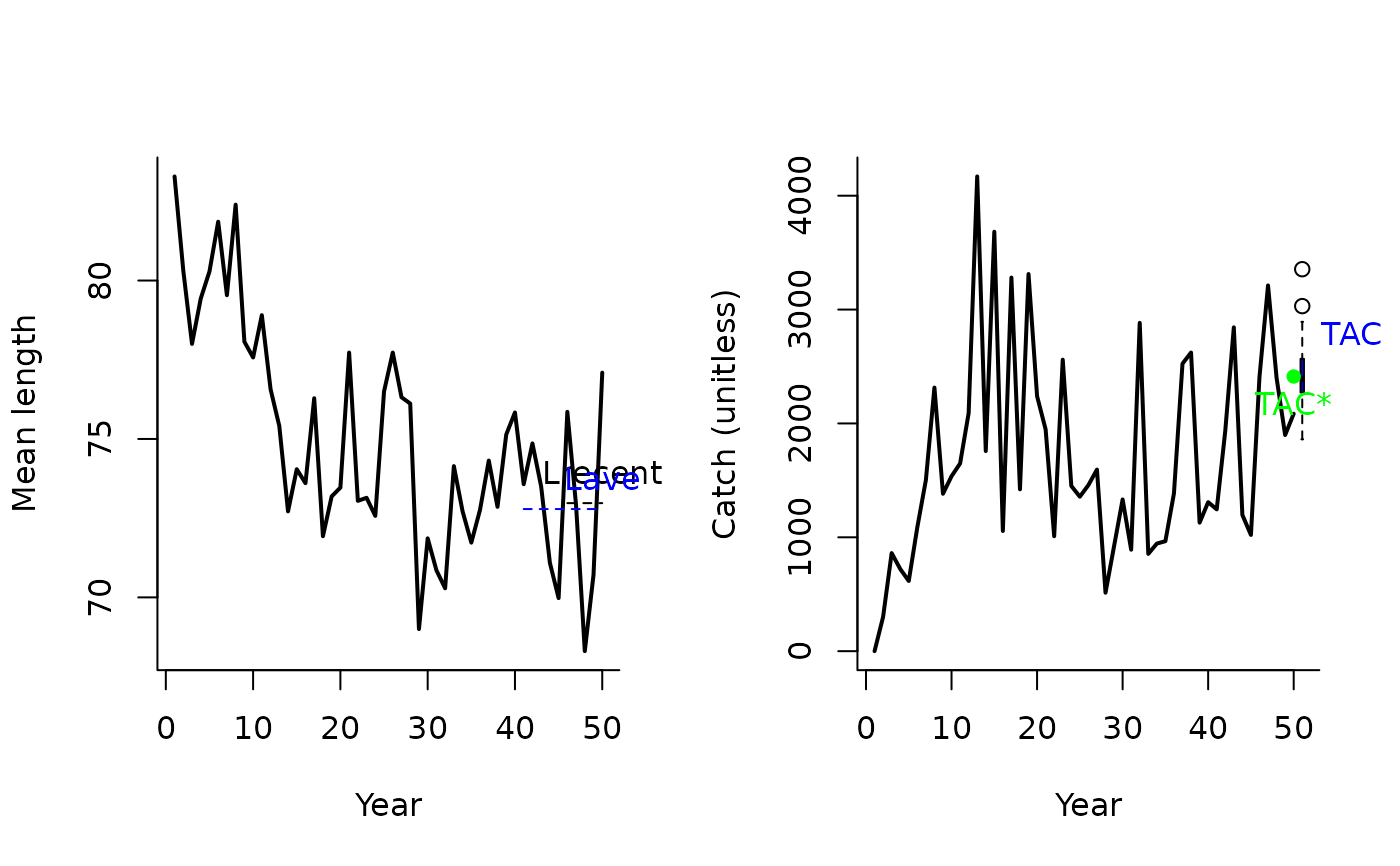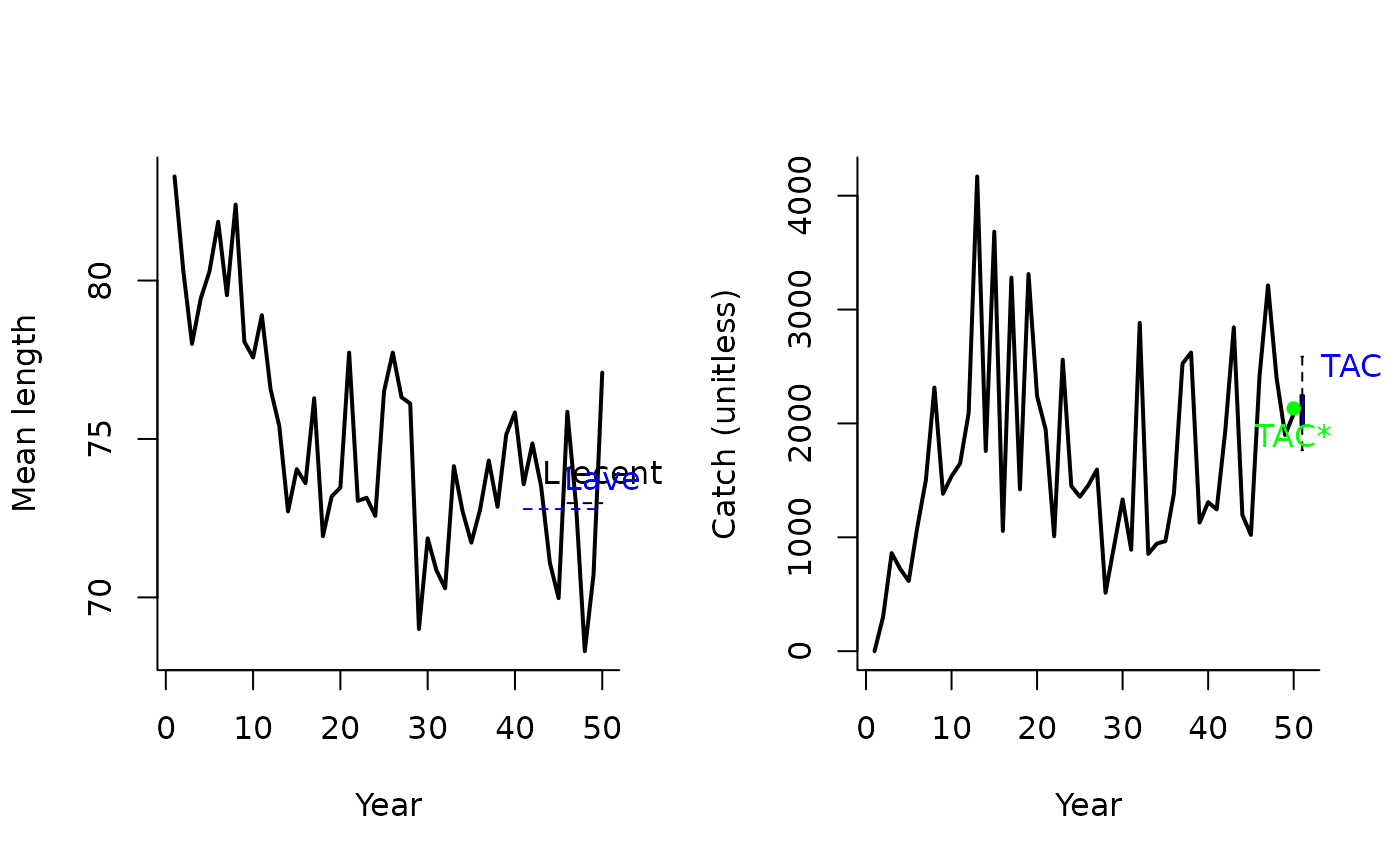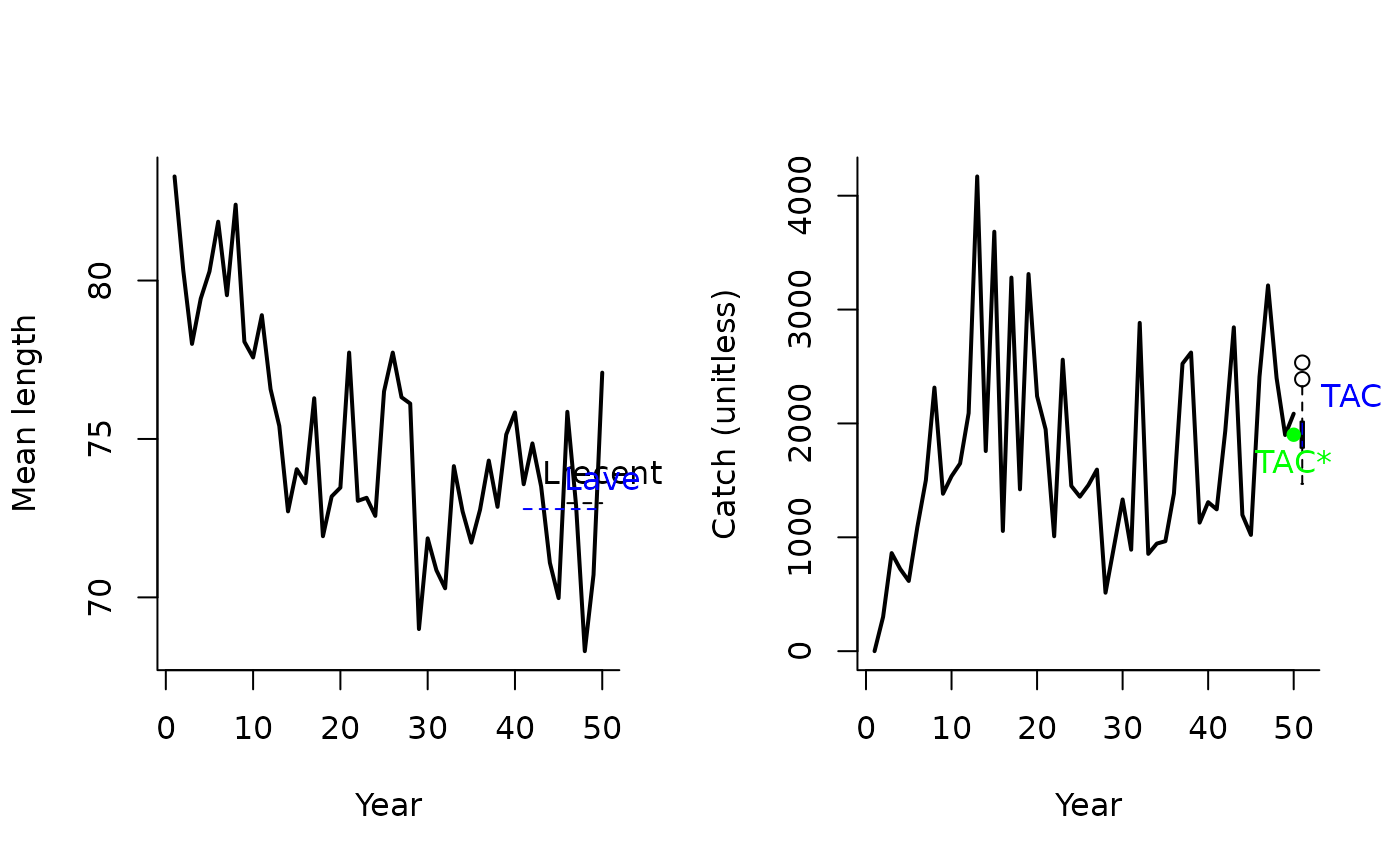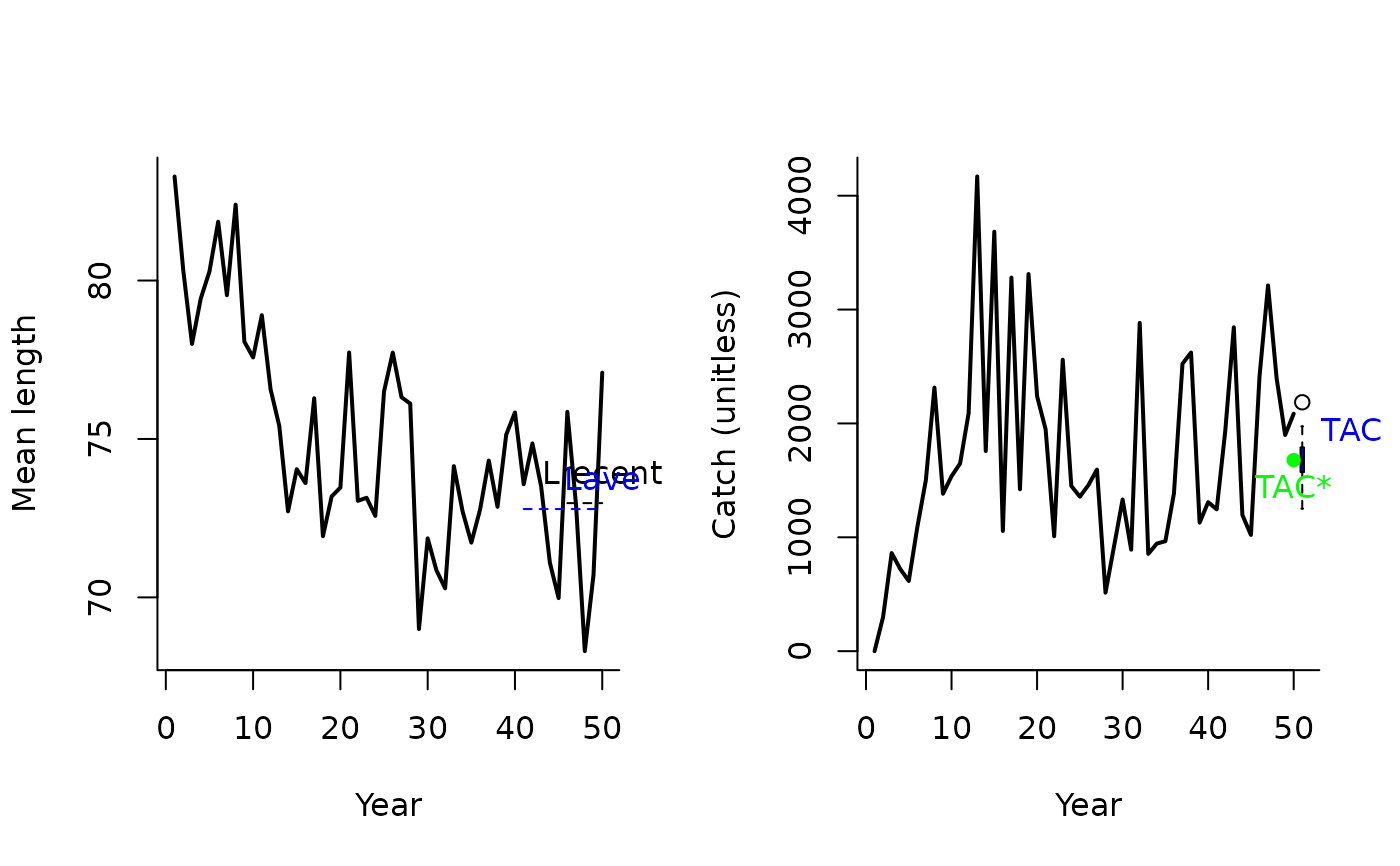A management procedure that incrementally adjusts the TAC according to the mean length of recent catches.

LstepCC1(
x,
Data,
reps = 100,
plot = FALSE,
yrsmth = 5,
xx = 0,
stepsz = 0.05,
llim = c(0.96, 0.98, 1.05)
)

LstepCC2(
x,
Data,
reps = 100,
plot = FALSE,
yrsmth = 5,
xx = 0.1,
stepsz = 0.05,
llim = c(0.96, 0.98, 1.05)
)

LstepCC3(
x,
Data,
reps = 100,
plot = FALSE,
yrsmth = 5,
xx = 0.2,
stepsz = 0.05,
llim = c(0.96, 0.98, 1.05)
)

LstepCC4(
x,
Data,
reps = 100,
plot = FALSE,
yrsmth = 5,
xx = 0.3,
stepsz = 0.05,
llim = c(0.96, 0.98, 1.05)
)

## Arguments

x

A position in the data object

Data

A data object

reps

The number of stochastic samples of the MP recommendation(s)

plot

Logical. Show the plot?

yrsmth

Years over which to calculate mean length.

xx

Parameter controlling the fraction of mean catch to start using in first year

stepsz

Parameter controlling the size of update increment in TAC or effort.

llim

A vector of length reference points that determine the conditions for increasing, maintaining or reducing the TAC or effort.

## Value

An object of class Rec-class with the TAC slot populated with a numeric vector of length reps

## Details

The TAC is calculated as: $$\textrm{TAC} = \left\{\begin{array}{ll} \textrm{TAC}^* - 2 S\textrm{TAC}^* & \textrm{if } r < 0.96 \\ \textrm{TAC}^* - S \textrm{TAC}^* & \textrm{if } r < 0.98 \\ \textrm{TAC}^* & \textrm{if } > 1.058 \\ \end{array}\right.$$ where $$\textrm{TAC}^*$$ is (1-xx) times average catch in the first year, and previous catch in all projection years, $$S$$ is step-size determined by stepsz, and $$r$$ is the ratio of $$L_\textrm{recent}$$ and $$L_\textrm{ave}$$ which are mean length over the most recent yrsmth years and 2 x yrsmth historical years respectively.

The conditions are specified in the llim argument to the function.

## Functions

• LstepCC1: The least biologically precautionary TAC-based MP.

• LstepCC2: More biologically precautionary than LstepCC1 (xx = 0.1)

• LstepCC3: More biologically precautionary than LstepCC2 (xx = 0.2)

• LstepCC4: The most precautionary TAC-based MP.

## Required Data

See Data-class for information on the Data object

LstepCC1: Cat, LHYear, ML, Year

LstepCC2: Cat, LHYear, ML, Year

LstepCC3: Cat, LHYear, ML, Year

LstepCC4: Cat, LHYear, ML, Year

## Rendered Equations

See Online Documentation for correctly rendered equations

T. Carruthers

## Examples

LstepCC1(1, Data=MSEtool::SimulatedData, plot=TRUE)#> TAC (median)
#>     2397.864

LstepCC2(1, Data=MSEtool::SimulatedData, plot=TRUE)#> TAC (median)
#>     2173.559
LstepCC3(1, Data=MSEtool::SimulatedData, plot=TRUE)#> TAC (median)
#>     1924.703
LstepCC4(1, Data=MSEtool::SimulatedData, plot=TRUE)#> TAC (median)
#>     1662.791